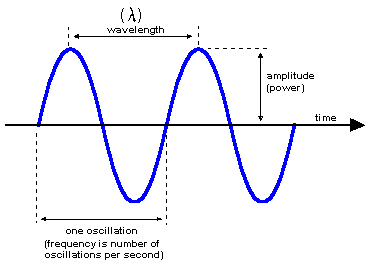# Definition: wavelength

The distance between crests of a wave. The wavelength determines the nature of the various forms of radiant energy that comprise the electromagnetic spectrum. For electromagnetic waves, the wavelength in meters is computed by the speed of light divided by frequency (300,000,000/Hz). For sound waves, the wavelength is determined by 335/Hz. See optical bands.Length of a Wave
The wavelength is the distance between crests. The higher the frequency, the shorter the wavelength.Wavelengths of Visible Light
The wavelengths of light that humans can see range from approximately 400 nm to 750 nm.